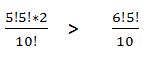# Time, Distance & Inequalities Questions From SBI PO Mains: 2017

### Question 1:

There are five couples attending a party ( all couple has a husband and a wife ).The probability associated with each quantity below with condition is provided

Quantity I : The probability associated with arranging the couples such that male and females are alternate
Quantity II : the probability associated with the case when all male are together.

a) quantity I > quantity II
b)quantity II > quantity I
c) quantity I quantity II
d) quantity I quantity II
e)no relation can be established or quantity I = quantity II

### Question 2:

There are 65 cards (numbered 1,2,3,4, …… 65) in a box. Two cards are picked at random .
Quantity I: Probability that both cards show 2 digit numbers which increase by 36 when its digit are reversed
Quantity II: Probability that both cards show a number divisible by 8 but not 16

a) quantity I > quantity II
b)quantity II > quantity I
c) quantity I quantity II
d) quantity I quantity II
e)no relation can be established or quantity I = quantity II

### Question 3:

There are two stations P and Q which are 516 km apart, Train A starts from station P at 9.45 am and goes towards Q and train B starts from Q 30min after train A towards P and they meet at 12.55. Train B speed is 1.5 times train A. Then  Relative speed of A to B in m/s

a) 45m/s
b)55m/s
c)50m/s
d)60 m/s
e)none

### Question 4:

There are two stations P and Q which are 516 km apart, Train A starts from station P at 9.45 am and goes towards Q and train B starts from Q 30min after train A towards P and they meet at 12.55. Train B speed is 1.5 times train A. Then  If B travels in the same direction as A. what time B will take to catch train A ?

a) 45 min later
b) 50 min later
c) 60 min later
d) 35min later
e)none

#### 1) a

Quantity I :Quantity II : Males have to be together , so we can take them as one unit while arranging both male and females ( but they will arrange internally among themselves )
they arrange themselves in 6 places in 6! Ways x 5! ( males arrangement )

Quantity II :Comparing both quantityQuantity I is greater than II

#### 2) aQuantity I : 5c₂ / 65 c₂
Qunatity II : numbers divisible by 8 will be 8, 16, 24,32,40,48,56 ,64
But numbers divisible by 16 should not be taken so 16 ,32 ,48,64 are eliminated
Only four numbers i.e. 8 ,24, 40 , 56 are considered , we have to choose any two out of these
So 4C₂ / 65C₂ is the quantity II
Clearly :Quantity I > Quantity II (a) is the right answer.

#### 3) cGiven speed ratio x : 1.5 x
Relative speed(opposite direction) = (x + y) km/hr = ( 1 x+ 1.5 x = 2.5 x )
When opposite direction given ,we can make starting time same by chossing either A or B ‘s timing
Train A starts before 30 min , and covers 1/2 * X In that duration and the rest distance will be
516 - 1/2 * X
From B’s side :
Distance / relative speed = time
(516 - 1/2 * X ) / 2.5x = 8/3 ( 2hrs. 40 min )
X = 12* 6
We need to find what is 2.5 x , so relative speed = 2.5 * 12*6 * (as asked in meters)
Relative speed = 50 m/s

#### 4) c

If both travel in same direction then relative speed will be subtraction of both speeds
Relative speed = 1.5x – 1x = 0.5x
B will have to takeover by travelling ½ x
Time taken by B to overtake A = ½ x / 0.5x = 1hour = 60 min later .